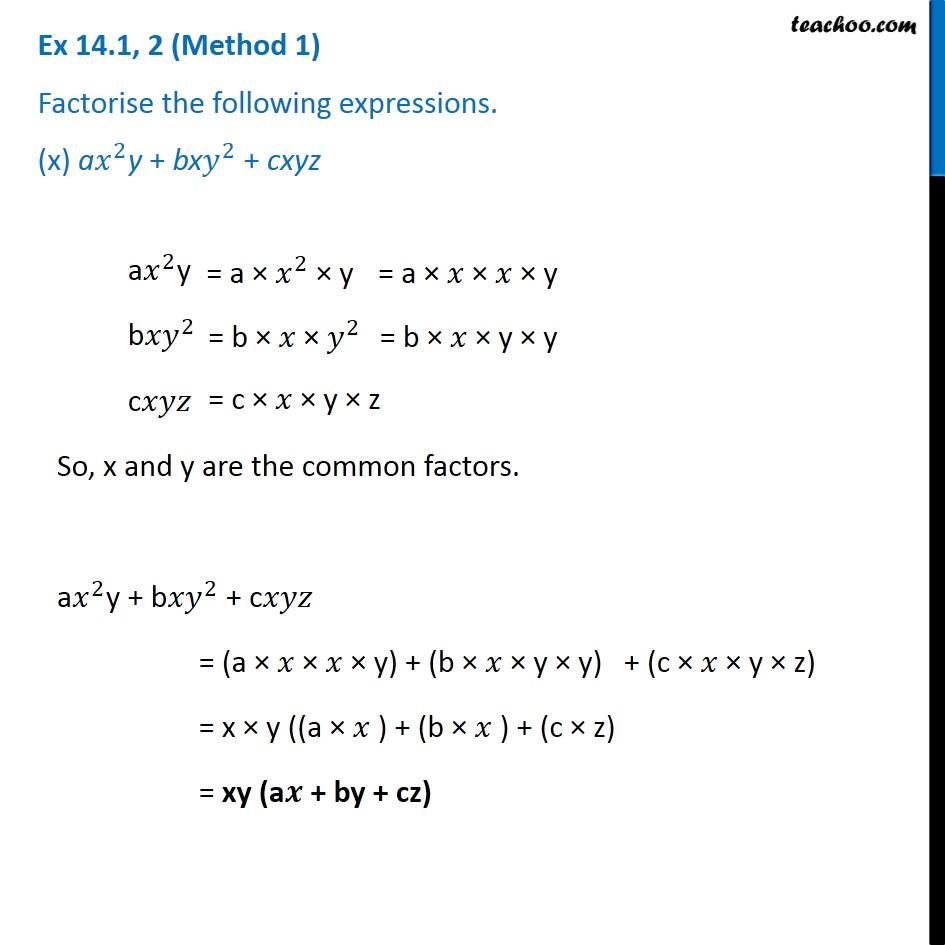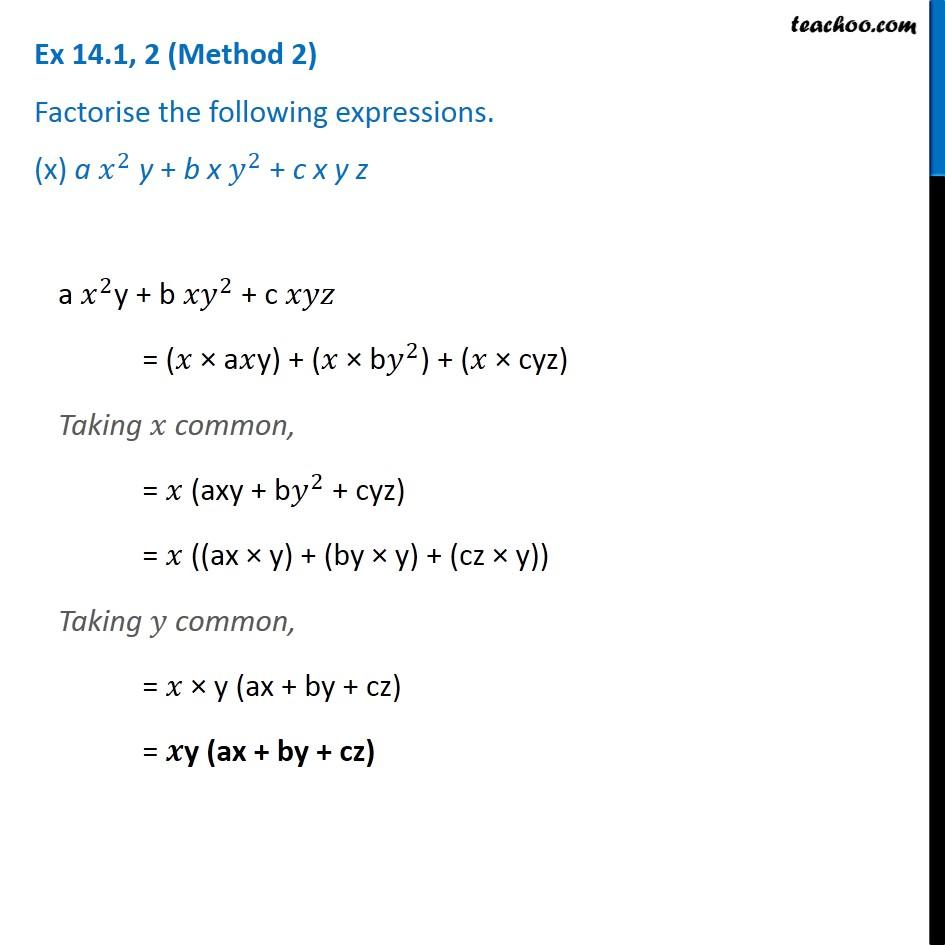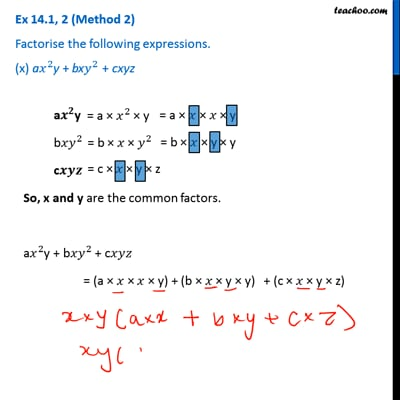Factorisation using common factors

Chapter 12 Class 8 Factorisation
Concept wiseThis video is only available for Teachoo black usersThis video is only available for Teachoo black users

Learn in your speed, with individual attention - Teachoo Maths 1-on-1 Class

### Transcript

Ex 12.1, 2 (Method 1) Factorise the following expressions. (x) a𝑥^2y + bx𝑦^2 + cxyz a𝑥^2y = a × 𝑥^2 × y = a × 𝑥 × 𝑥 × y b𝑥𝑦^2 = b × 𝑥 × 𝑦^2 = b × 𝑥 × y × y c𝑥𝑦𝑧 = c × 𝑥 × y × z So, x and y are the common factors. a𝑥^2y + b〖𝑥𝑦〗^2 + c𝑥𝑦𝑧 = (a × 𝑥 × 𝑥 × y) + (b × 𝑥 × y × y) + (c × 𝑥 × y × z) = x × y ((a × 𝑥 ) + (b × 𝑥 ) + (c × z) = xy (a𝒙 + by + cz)